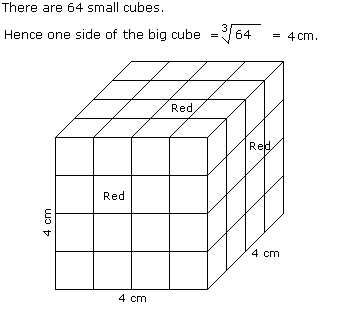# Verbal Reasoning - Cube and Cuboid - Discussion

The following questions are based on the information given below:

1. All the faces of cubes are painted with red colour.
2. The cubes is cut into 64 equal small cubes.

2.

How many small cubes have no faces coloured ?

 [A]. 24 [B]. 8 [C]. 16 [D]. 0

Explanation:Number of small cubes having only one faces coloured = (x - 2)3

Here, x = side of big cube / side of small cube

x = 4 /1

x = 4

Required number = (4 -2)3

= 8

 Fathima said: (Jun 21, 2013) I can't understand. But they gave the condition like "All the faces of cubes are painted with red colour". Please explain me. Thanks in Advance.

 Alen said: (Sep 28, 2013) Its talks about the small CUBES which is inside the BIG CUBE, a 2X2X2 cube will be there inside the big cube remain unpainted !hence the answer 8. hope its helpful :)

 Anjali said: (Jun 8, 2020) The number of cubes with 0 side painted = (x-2)^3. As x=4(∛64) ,ans is (4-2)^3=8.